Lightmap Storage Question submitted by (06 June 2000)Return to The Archives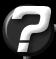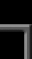I'd like to implement light mapping in my 3D-Engine. Light mapping is not my problem, but the storage of the Lightmaps. Everybody says that you should use a 16:1 ratio for your lightmaps. I think that's not good for every poly because your lightmaps are stretch for every poly. If you have a big poly then your lightmap texels are very big, or if you have a small poly then your lightmap texels are very small. You can calculate an individual lightmap ratio for every poly. Primarily I would use Memory-Banks of 256x256, and a lightmap ratio of 16.1 so I can put 256 lightmaps in one bank. But if I calculate an individual lightmap ratio for every poly then I can get or better I get different lightmap ratios. So I can't put 256 in one Memory-Bank or maybe the ratio is greater as 256 than I can't create a lightmap in a Memory-Bank. I experimented with Quake3Arena and Quake and I found out that the lightmaps of all polys (small polys, big polys) consist of the same sized texels. How do they perform this? I think I need a sort algorithm, to find the best structure of the different sized lightmaps in the Memory-Banks. Is the way the right?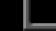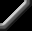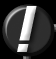Your solution is hidden in the mapping, not the storage of those lightmaps. I've covered this a couple times before, but not to my satisfaction. Let's try this again...

Ideally, you want your lightmaps to be relative to space, not the size of the polygons they cover. In other words, you want your light map resolutions to be "one texel equals one square centimeter, regardless of how big the polygon is" or something similar. This is called "texel density". There is an inherent problem by going with this, and that is the problem of a polygon that is so large that it requires a texture larger than your maximum texture size (for example, 256x256.) The solution to this is to find these situations and split the polygon into multiple pieces, each of which being no larger than your largest texture size. I know that at least KAGE & Quake both do this. I won't cover this here, but I will cover the mapping process. Once you understand the mapping process, you should be able to figure out the process to split the problem polygons that extend past the maximum size of a lightmap.

The trick is to use world-space coordinates to do your mapping.

By using world-space coordinates, you also solve another tricky problem. As far as I can tell, this is the only way to solve this problem properly. The problem I'm referring to is the problem of lightmap seams between abutting polygons. Unless you get the lightmap texels lined up perfectly between two polygons, you'll get seams. This problem is easily solved for boxes, but what about non-orthogonal geometry? What about light mapping a sphere? If you line up the lightmaps on two polygons from a sphere, you'll throw off the alignment of other neighboring polygon's lightmaps.

The trick is picking the proper UV coordinates for your lightmaps. This process is actually quite simple and automatic process of planar mapping from the orthogonal axes.

Think of it as if you're viewing a polygon that is directly facing the camera. If you were to gradually rotate this polygon away from the camera, it would occupy less and less screen space, until it was infinitely thin (i.e. at a 90-degree angle to the camera.) We want to map the polygon from the axis that sees the "largest view" of the polygon. By doing this, we get the most detail mapped onto our polygon with as little "stretching" as possible. Stretching is bad, yes, but in this case, it's necessary. It's this stretching that allows us to properly map a sphere with the texture and avoid the seams. Without stretching the texture at least a little, we would not be able to map a sphere with a series of flat textures that all line up at the edges of the polygons. But we want to minimize the stretching as much as possible, so we apply our mapping from the axis that "sees" the most of our polygon (i.e. the polygon's primary axis.)

By primary axis, I mean if the polygon faces mostly forward, mostly up, or mostly to the right (or the inverse of any of these.) To do this, we simply need to get the polygon's normal (x, y & z) and find the largest value. The largest value is the direction that the polygon primarily faces (i.e. it's primary axis.) So if the polygon's normal's X is larger than its Y or Z, then the polygon primarily faces along the X-axis.

To extend this, let's consider a polygon that faces directly along the X-axis. This polygon will have no deviation from vertex to vertex in the X direction. In other words, the X coordinate of all vertices in this polygon will all be equal (this polygon lies flat on the X plane.)

Extending this even further, we find that if we were to throw away the X coordinate of the polygon's vertices (remember, they're all equal in this case) we're left with a 2D polygon, using the Y and Z components of each vertex. If you were to visit each vertex and copy these Y and Z world-space coordinates into its U and V coordinates for the polygon's lightmap, you've just mapped the polygon in a planar fashion along the X-axis.

But not all polygons face directly along their primary axis. The polygons that are slightly rotated away from their primary axis (up to 45 degrees) will have stretched texels. But this stretching is necessary. And the stretching is never more than a 2:1 ratio at it's worst. This is actually, hard to notice. Did you noticed this when looking at Q3Arena? It's there.

We're not done yet. We've still got two problems to solve. First, we've simply copied the Y and Z world-space components over. This gives us a 1:1 correlation from world-space density to lightmap density. In other words, if one unit in our world space were one meter, this would mean that a lightmap texel would be one square meter in size. So we need a way to scale it. I do this by simply multiplying the resulting lightmapping UVs by a scalar. If we wanted our final lightmap density to be one square centimeter per lightmap texel, then we would divide our UVs by 100.0 (or, multiply by 0.01) since one centimeter is 1/100th of a meter. This scales our lightmaps to be the density that we want. By applying this process to every polygon in a scene, we get automatic lightmap mapping of the entire scene that will never have a single seam in the lightmaps.

Here's a pseudo-code snippet of the process up to this point:

 ``` void Polygon::calcLightMapCoords(double density) { // Calculate the |Normal| of the polygon, using // the polygon's normal (this does an ABS() on each // element of the normal) Vector absNorm = normal.abs(); // Does the polygon primarily face along the X-axis? if (absNorm.x >= absNorm.y && absNorm.x >= absNorm.z) { for (each vertex in polygon) { vertex.lmap.u = vertex.world.z * density; vertex.lmap.v = -vertex.world.y * density; } } // Does the polygon primarily face along the Y-axis? else if (absNorm.y >= absNorm.x && absNorm.y >= absNorm.z) { for (each vertex in polygon) { vertex.lmap.u = vertex.world.x * density; vertex.lmap.v = -vertex.world.z * density; } } // The polygon primarily face along the Z-axis else { for (each vertex in polygon) { vertex.lmap.u = vertex.world.x * density; vertex.lmap.v = -vertex.world.y * density; } } } ```

But we're still not done... Since we're just copying in world-space coordinates (and scaling them), we will most likely end up with UVs that are way out of range. We might end up with UVs for a single polygon that range from [1000-1004] in U and [6000-6100] in V. The size of this map is only 4x100, but the values are way out of range, so we need to translate them. This is as simple as finding the minimum U and minimum V and subtracting that from the rest. In this example, we would subtract 1000 from all the Us and we would subtract 6000 from all the Vs. This gives us a new set of ranges: [0-4] in U and [0-100] in V. Those are manageable. If our ranges ever exceed 256 (or your maximum texture size) then this is where you'll need to split the polygon.

Are we done yet? Nope. When we subtract the minimum values from our texels, we translate the upper-left corner of our lightmap texture to be relative [0,0]. But we didn't take the floating point precision into account. If, for example, our range in U was actually [1000.5-1004.5], then we've placed the center of that texel at [0,0]. This is not what we want. Also, we need to work on a fixed grid (as opposed to a floating-point grid) to avoid those seams. So before we do the subtraction, we simply need to run the minimum U and V through floor(). This aligns our texels on a 2-Dimensional grid on it's primary axis. This grid is what helps us avoid the seams for neighboring polygons - they all map to this same grid as a reference point.

When people say to use a mapping of 16:1, they mean that you want a single lightmap texel to be equal to a 16x16 grid of texture texels. But this only works if your textures have a similar world-space mapping technique. This technique works for textures as well, just pass in a different density (for 16:1 mapping, use a density that is 16 times greater than the one you use for your lightmap UVs.)

I recommend using this technique for textures and lightmaps. It's useful when trying to avoid seams in your textures as well as your lightmaps. It also keeps things manageable. I've had many cases where an artist mapped a surface with a texture that was so dense, it required over 100MB of surface caching space (remember the old days of software surface caching?) I was forced to use this technique on my textures to avoid this problem. By using this on textures, I found that everything has a uniform texture density (this makes for some good-looking results.) Of course, you'll want to provide a method for adjusting the automatically mapped textures, so you can line your textures up with your geometry. But it's the density that matters, and this technique gives you a great starting point.Response provided by Paul Nettle